# ABSOLUTE Function in Excel (ABS)

Returns the absolute value of a number

## What is the ABSOLUTE Function in Excel (ABS)?

The ABSOLUTE function in Excel returns the absolute value of a number. The function converts negative numbers to positive numbers while positive numbers remain unaffected.

### Formula

ABSOLUTE Value = ABS(number)

Where number is the numeric value for which we need to calculate the Absolute value.

### How to use the ABSOLUTE Function in Excel?

Let’s take a series of numbers to understand how this function can be used.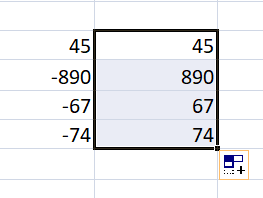In the screenshot above, we are given a series of numbers. When we use the ABSOLUTE function, we get the following results:

1. For positive numbers, we get the same result. So 45 is returned as 45.
2. For negative numbers, the function returns absolute numbers. So for -890, -67, -74, we got 890, 67, 74.

### Examples of the ABSOLUTE Function in Excel

For our analysis, we want the difference between Series A and Series B as given below. Ideally, if you subtract Series A from Series B you might get negative numbers depending on the values. However, if you want absolute numbers in this scenario, we can use the ABSOLUTE function.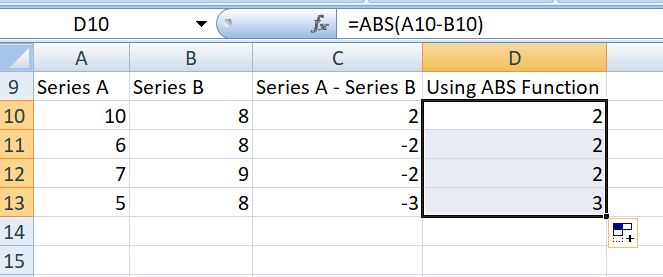The results returned using ABSOLUTE function would be absolute numbers. So ABS can be combined with other functions such as SUM, MAX, MIN, AVERAGE, etc. to calculate the absolute value for positive and negative numbers in Excel spreadsheets.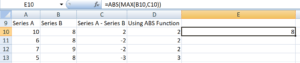Let’s see a few examples of how ABS can be used with other Excel Functions.

#### 1. SUMIF and ABS

We all are aware that SUMIF would sum up values if certain criteria within the range given are met. Let’s assume we’ve been given a few numbers in Column A and Column B as below: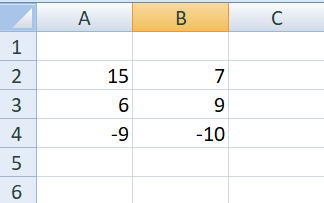Now, I wish to subtract all negative numbers in Column B from all positive numbers of Column A. I want the result to be an absolute number. So I can use the ABS function along with SUMIF in the following manner: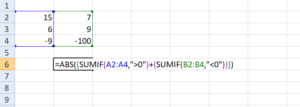The result is 79. Excel added 15 and 6 from Column A and subtracted 100 from Column B to give us 79, as we used ABS function instead of -79.

#### 2. SUM ARRAY Formula and ABS Function

The Excel array formulas help us to do multiple calculations for a given array or column of values. We can use SUM ARRAY along with ABS to get the absolute value of a series of numbers in column or row. Suppose we are given a few numbers as below, so in this scenario, the SUM array formula for absolute values would be =SUM(ABS(A2:A6)).

Now, select cell A7 in your spreadsheet, and enter the formula ‘=SUM(ABS(A2:A6))’. After entering the formula in cell A7, press “Ctrl + Shift + Enter”. Once we do this, the formula will have {} brackets around it as shown in the screenshot below.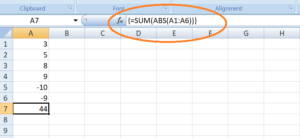As seen in the screenshot above, the array formula also returned the value 44 in cell A7, which is the absolute value of the data entered in cells A2:A6.

#### 3. SUMPRODUCT Formula and ABS Function

The SUMPRODUCT function allows us to include the ABS function to provide absolute numbers. Suppose we are given the following data. If we would just use the SUMPRODUCT formula, we would get a negative number as shown below: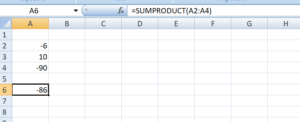However, using ABS Function, we can get the absolute number as a result. The formula to be used would be: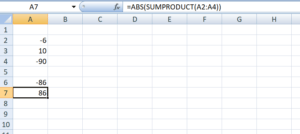### ABS as a VBA Function

If we wish to use the ABSOLUTE function in Excel VBA code, it can be used in the following manner. Let’s assume I need ABS of -600 so the code would be:

Dim LNumber As Double

LNumber = ABS(-600)

Now in the above code, the variable known as LNumber would now contain the value of 600.

### Free Excel Course

If you want to learn more Excel functions with your own online instructor, check out CFI’s Free Excel Crash Course!  You’ll get step by step tutorials and demonstrations on how to become an Excel power user.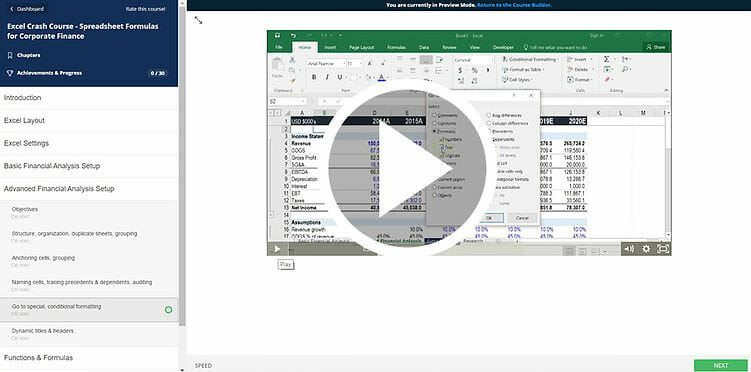Thanks for reading CFI’s guide to important Excel functions! By taking the time to learn and master these functions, you’ll significantly speed up your financial analysis. To learn more, check out these additional CFI resources:

• Excel Functions for Finance